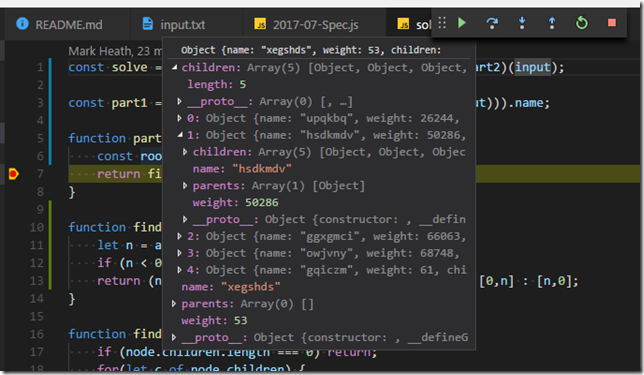Today’s Advent of Code challenge was a little trickier than previous ones, and I didn’t help myself by making a couple of silly mistakes on part 2. It did however provide an opportunity to use some techniques we’ve not yet put to use including regular expressions, recursion, depth first searches and debugging node.js in Visual Studio Code.

### Parsing input with regex

First of all, we needed to parse input that looked like this:

``````ktlj (57)
fwft (72) -> ktlj, cntj, xhth
qoyq (66)
padx (45) -> pbga, havc, qoyq
``````

JavaScript has a built-in regex syntax, that is more straightforward to work with than the .NET RegEx class. By calling `exec` on my regex I can then easily access the matched groups to parse the input into a list of “programs”

``````const parseInput = input => input.map(x =>
/(\w+) \((\d+)\)( -> ([\w, ]+))?/.exec(x))
.map(g => ({name:g,weight:Number(g),children:g?g.split(', '):[],parents:[] }))
``````

### Building the tree

I then created a simple function to update my list to properly link to all children (rather than just having an array of their names), and include backwards links to parents. I used the ES6 `Map` again as an easy way to create a lookup of nodes by name.

``````function buildTree(programs) {
let nodes = new Map(programs.map(p => [p.name,p]));

for(let p of programs) {
p.children = p.children.map(c => nodes.get(c))
for(let c of p.children) {
c.parents.push(nodes.get(p.name))
}
}
return programs;
}
``````

One gotcha I hit with `Map` is that you must use `get` rather than square brackets syntax to perform lookups.### Finding the root

The solution to part 1 was to find the root of the tree – i.e. the node with no parents. This could easily be done with the `find` method on `Array`:

``````function findRoot(programs) {
return programs.find(p => p.parents.length === 0);
}
``````

### Weighing nodes

For part 2, we needed the ability to calculate a node’s “weight”. This meant adding its own weight to the weights of all its children. This can be done simply with recursively calling to get the weight of our children, summing them together with `reduce`.

``````function getNodeWeight(node) {
return node.weight + node.children.reduce((a,b) => a + getNodeWeight(b),0)
}
``````

### Finding the unbalanced node

The main challenge of part 2 was to find the “unbalanced” node and return the new weight it should be given. I chose to start from the root node, and again recursively look for an “unbalanced” node. An unbalanced node is one where all of its children don’t have the same weight.

My solution is below, but I made two mistakes that slowed me down. First, this needed to be a depth-first search. In other words, I must first check if my children are unbalanced before checking if I am unbalanced. So the function starts by recursively checking the children and only then checking whether this node is unbalanced.

``````function findUnbalancedNode(node) {
if (node.children.length === 0) return;
for(let c of node.children) {
let unbalanced = findUnbalancedNode(c);
if (unbalanced) return unbalanced;
}

let weights = node.children.map(getNodeWeight);
let [differentIndex,correctIndex] = findDifferentIndex(weights);
if (differentIndex < 0) { // all same
return;
}
else {
let difference = weights[differentIndex] - weights[correctIndex];
let differentNode = node.children[differentIndex];
return {differentNode,correctWeight:differentNode.weight - difference};
}
}
``````

My second mistake was in the `findDifferentIndex` function. The premise is simple – in an array of numbers where one maybe differs from the others, find the index of the different one. It sounds simple, but I managed to get it wrong resulting in me submitting some wrong answers for the first time this year. Here’s a working version (that I really should clean up further now its fixed)

``````function findDifferentIndex(arr) {
let n = arr.findIndex(w => w !== arr)
if (n < 0) return [-1];
return (n === 1 && arr[n] === arr[arr.length - 1]) ? [0,n] : [n,0];
}
``````

This is the type of mistake that a good suite of unit tests should find. For example, here are some Jasmine tests that had I written them up front would have found my mistake immediately:

``````it ("finds different index if different is first", function() {
expect(findDifferentIndex([2,1,1,1])).toEqual([0,1])
})

it ("finds different index if different is last", function() {
expect(findDifferentIndex([1,1,1,2])).toEqual([3,0])
})

it ("finds different index if different is second", function() {
expect(findDifferentIndex([1,2,1,1])).toEqual([1,0])
})
``````

### Debugging Node in VS Code

My troubles getting part 2 correct meant I had my first opportunity to try out debugging node in Visual Studio Code. I was very impressed with how easy it was. Simply add a breakpoint, hit F5 and VS Code automatically launched `index.js` and attached a debugger. It really was very straightforward and its impressive how VS Code is good enough to be an IDE replacement in many scenarios.As usual you can explore my full solution to today’s puzzle on GitHub.